Remainder Theoram

Chapter 2 Class 9 Polynomials (Term 2)
Concept wise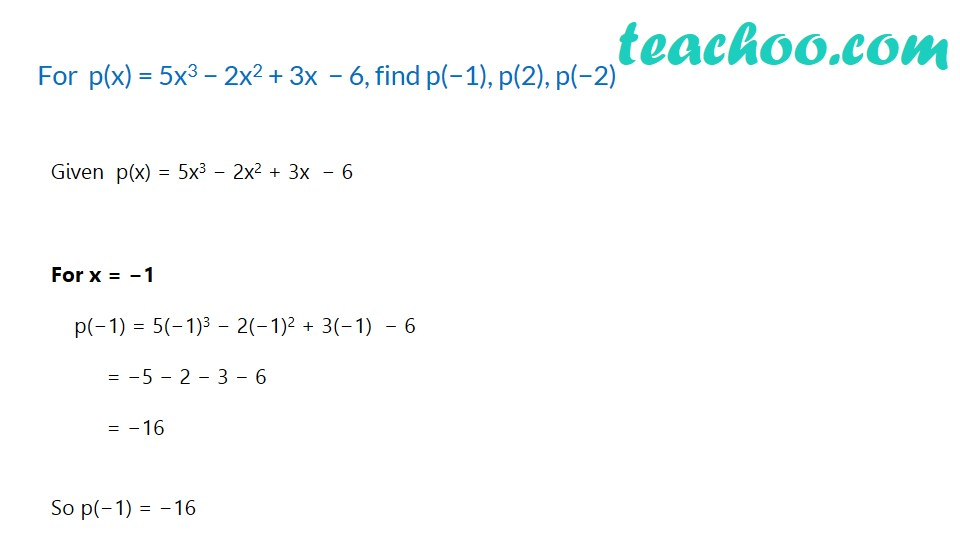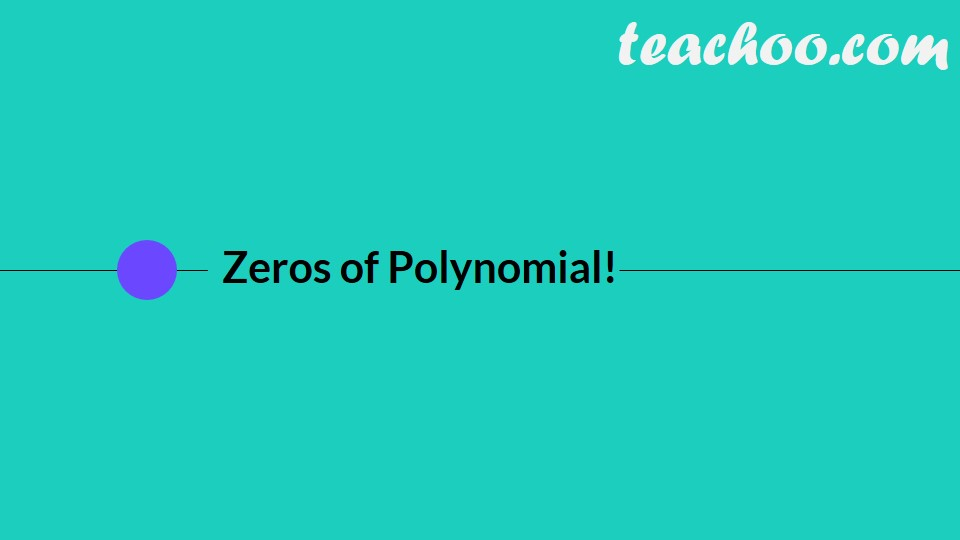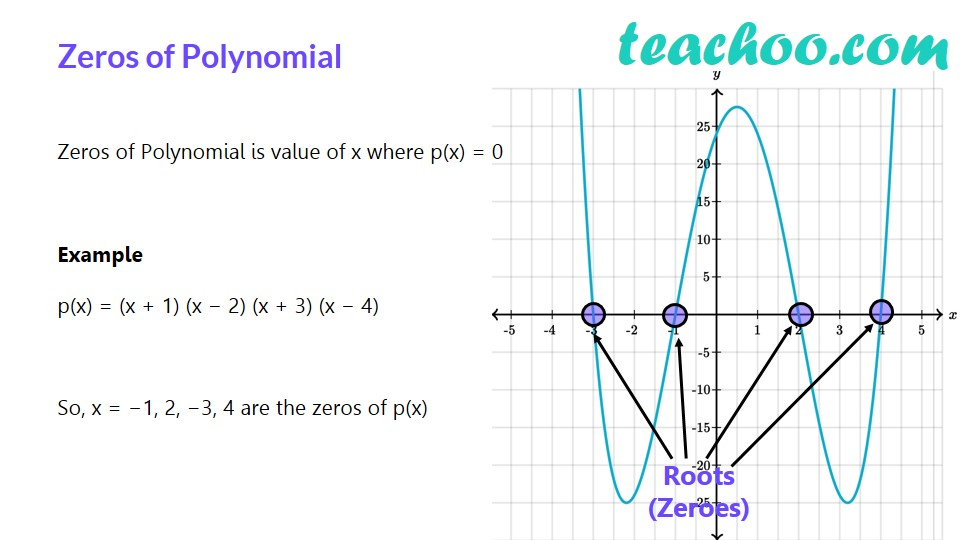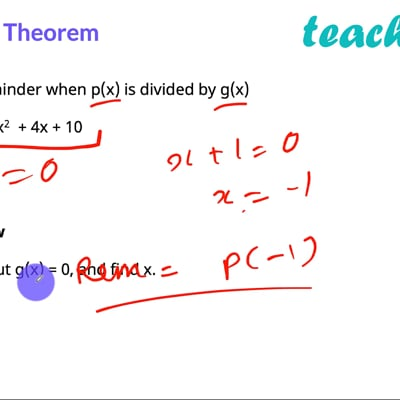This video is only available for Teachoo black users

Let us find remainder when p(x) is divided by g(x)

p(x) = x 3 − 3x 2   + 4x + 10

g(x) = x + 1

## By Long Division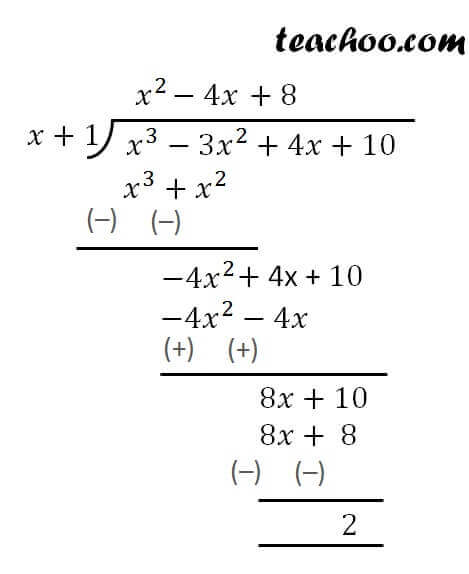So, Remainder = 2

There is another method to find remainder by Remainder Theorem

## Remainder Theorem

In remainder theorem

1. We put g(x) = 0, and find x.
2. Let x = a
3. Put a in p(x)
4. Remainder = p(a)

So, in our example

If  p(x) = x 3 − 3x 2   + 4x + 10 is divided by g(x) = x + 1

Putting g(x) = 0

x + 1 = 0

x =  − 1

So, Remainder = p(−1)

= (−1) 3   − 3(−1) 2 + 4(−1) + 10

= (−1)  − 3 × 1  − 4 + 10

= −1 − 3 − 4 + 10

= 2

So, Remainder = 2

### Transcript

Dividing two numbersQuotient Divisor Dividend Remainder Which can be rewritten as a sum like this: Division Algorithm is Dividend = Divisor × Quotient + Remainder Quotient Divisor Dividend Remainder Dividing two PolynomialsLet’s divide 3x2 + x − 1 by 1 + x We can write Dividend = Divisor × Quotient + Remainder 3x2 + x – 1 = (x + 1) (3x – 2) + 1 What if…We divide 3x2 + x − 1 by x + 1 So, Dividend = Divisor × Quotient + Remainder 3x2 + x – 1 = (x + 1) × Quotient + Remainder Putting x = −1 3(−1)2 + (−1) – 1 = (−1 + 1) × Quotient + Remainder 1 = Remainder Remainder = 1